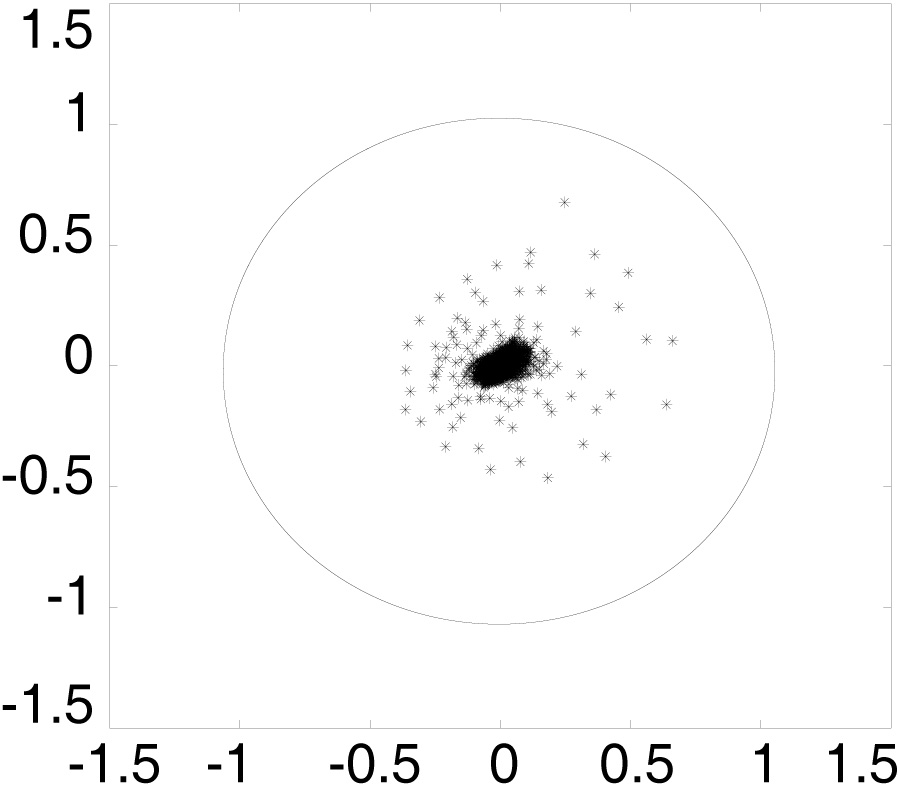# Vol. 97

Front:[PDF file] Back:[PDF file]
Latest Volume
All Volumes
All Issues
2009-10-19

#### A Numerical Method for Electromagnetic Scattering from Dielectric Rough Surfaces Based on the Stochastic Second Degree Method

By Yang Du and Bin Liu
Progress In Electromagnetics Research, Vol. 97, 327-342, 2009
doi:10.2528/PIER09092501

## Abstract

In this paper, we propose an iterative numerical approach based on the stochastic second degree (SSD) algorithm in combination with a new splitting of the impedance matrix to analyze electromagnetic scattering from 1-D dielectric rough surfaces. The embedded matrix-vector product is computed using the banded matrix iterative approach/canonical grid (BMIA/CAG) and the spectral acceleration (SA) technique. For Gaussian surface with Gaussian spectrum, through extensive numerical simulation, it is observed that for HH polarization, the proposed method is slightly less computationally efficient in terms of run time and number of iterations than its counterpart without the SSD algorithm. However, the proposed method obviously improves the convergence properties over its counterpart by changing cases from divergent to convergent when the rms height and rms slope are large. For VV polarization, the relative performance in terms of number of iterations of the proposed method shows appreciable improvement and becomes better starting from the rms slope of 0.55 uniformly across all rms heights. As far as the convergence properties are considered, the proposed method obviously improves over its counterpart for certain large rms slopes. In short, the proposed method demonstrates its superiority when dealing with truly rough surfaces.

## Citation

Yang Du and Bin Liu, "A Numerical Method for Electromagnetic Scattering from Dielectric Rough Surfaces Based on the Stochastic Second Degree Method," Progress In Electromagnetics Research, Vol. 97, 327-342, 2009.
doi:10.2528/PIER09092501
http://www.jpier.org/PIER/pier.php?paper=09092501

## References

1. Tsang, L., J. A. Kong, and R. T. Shin, Theory of Microwave Remote Sensing, Wiley-Interscience, New York, 1985.

2. Liang, D., P. Xu, L. Tsang, Z. Gui, and K.-S. Chen, "Electromagnetic scattering by rough surfaces with large heights and slopes with applications to microwave remote sensing of rough surface over layered media ," Progress In Electromagnetics Research, Vol. 95, 199-218, 2009.
doi:10.2528/PIER09071413

3. Zhou, L., L. Tsang, V. Jandhyala, and C.-T. Chen, "Studies on accuracy of numerical simulations of emission from rough ocean-like surfaces," IEEE Trans. Geoscience and Remote Sensing, Vol. 39, 1757-1763, 2001.
doi:10.1109/36.942554

4. Li, Z.-X., "Bistatic scattering from rough dielectric soil surface with a conducting object with arbitrary closed contour partially buried by using the FBM/SAA method ," Progress In Electromagnetics Research, Vol. 76, 253-274, 2007.
doi:10.2528/PIER07071501

5. Wang, M.-J., Z.-S. Wu, and Y.-L. Li, "Investigation on the scattering characteristics of Gaussian beam from two dimensional dielectric rough surfaces based on the Kirchho® approximation," Progress In Electromagnetics Research B, Vol. 4, 223-235, 2008.
doi:10.2528/PIERB08010903

6. Wang, R. and L. Guo, "Numerical simulations wave scattering from two-layered rough interface," Progress In Electromagnetics Research B, Vol. 10, 163-175, 2008.
doi:10.2528/PIERB08082903

7. Pak, K., L. Tsang, C. H. Chan, and J. T. Johnson, "Backscattering enhancement of vector electromagnetic waves from two-dimensional random rough surfaces based on Monte Carlo simulations," J. Opt. Soc. Amer. A, Opt. Image Sci., Vol. 12, 2491-2499, 1995.
doi:10.1364/JOSAA.12.002491

8. Du, Y., Y. L. Luo, and J. A. Kong, "Electromagnetic scattering from randomly rough surfaces using the stochastic second-degree method and the sparse matrix/canonical grid algorithm," IEEE Trans. Geoscience and Remote Sensing, Vol. 46, 2831-2839, 2008.
doi:10.1109/TGRS.2008.921211

9. Du, Y., J. C. Shi, Z. Y. Li, and J. A. Kong, "Analysing EM scattering from randomly rough surfaces using stochastic second-degree iterative method, sparse matrix algorithm and Chebyshev approximation," Electronics Letters, Vol. 45, 292-293, 2009.
doi:10.1049/el.2009.2543

10. Li, Q., C. H. Chan, and L. Tsang, "Monte-Carlo simulations ofwave scattering from lossy dielectric random rough surfaces using the physics-based two-grid method and canonical grid method," IEEE Trans. Antennas Propagat., Vol. 47, 752-763, 1999.
doi:10.1109/8.774140

11. Tsang, L., J. A. Kong, K. H. Ding, and C. O. Ao, Scattering of Electromagnetic Waves, Numerical Simulations, Vol. 2, Wiley-Interscience, New Jersey, 2001.

12. Tsang, L., C. H. Chan, K. Pak, and H. Sangani, "MonteCarlo simulations of large-scale problems of random rough surface scattering and applications to grazing incidence with the BMIA/canonical grid method," IEEE Trans. Antennas Propagat., Vol. 43, 851-859, 1995.
doi:10.1109/8.402205

13. Chen, K.-S., L. Tsang, and J.-C. Shi, "Microwave emission from two-dimensional inhomogeneous dielectric rough surfaces based on physics-based two-grid method," Progress In Electromagnetics Research, Vol. 67, 181-203, 2007.
doi:10.2528/PIER06082903

14. Holliday, D., L. L. DeRaad, and G. C. St-Cyr, "Forward-backward: A new method for computing low-grazing angle scattering," IEEE Trans. Antennas Propagat., Vol. 44, 722-729, 1996.
doi:10.1109/8.496263

15. Iodice, A., "Forward-backward method for scattering from dielectric rough surfaces," IEEE Trans. Antennas Propagat., Vol. 50, 901-911, 2002.
doi:10.1109/TAP.2002.800700

16. West, J. and J. M. Sturm, "On iterative approaches for electromagnetic rough-surface scattering problems," IEEE Trans. Antennas Propagat., Vol. 47, 1281-1288, 1999.
doi:10.1109/8.791944

17. Liu, B., Z. Y. Li, and Y. Du, "A fast numerical method for electromagnetic scattering from dielectric rough surfaces,", submitted for publication.

18. Chou, H.-T. and J. T. Johnson, "Formulation of forward-backward method using novel spectral acceleration for the modeling of scattering from impedance rough surfaces," IEEE Trans. Geosci. Remot. Sens., Vol. 38, 605-607, 2000.
doi:10.1109/36.823954

19. Chou, H.-T. and J. T. Johnson, "A novel acceleration algorithm for the computation of scattering from rough surfaces with the forward-backward method," Radio Sci., Vol. 33, 1277-1287, 1998.
doi:10.1029/98RS01888

20., De Pillis and J., "Faster convergence for iterative solutions to systems via three-part splittings," SIAM J. Numer. Anal., Vol. 15, 888-911, 1978.
doi:10.1137/0715058